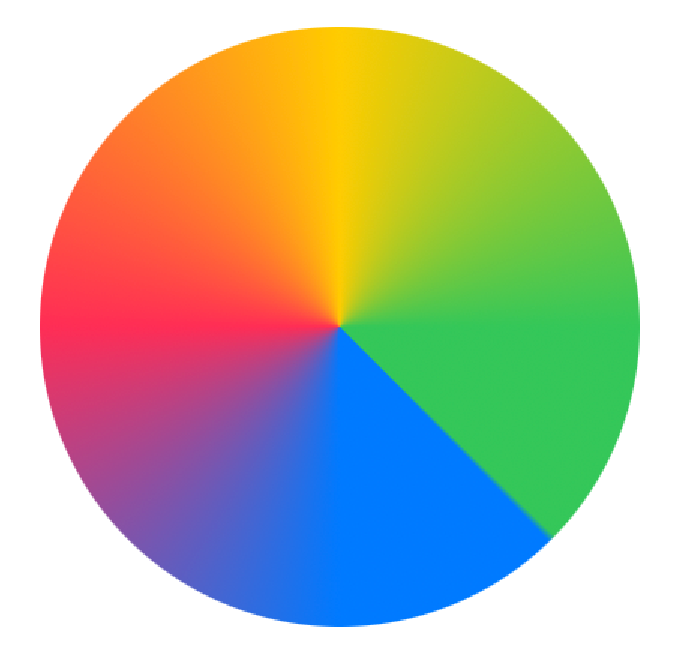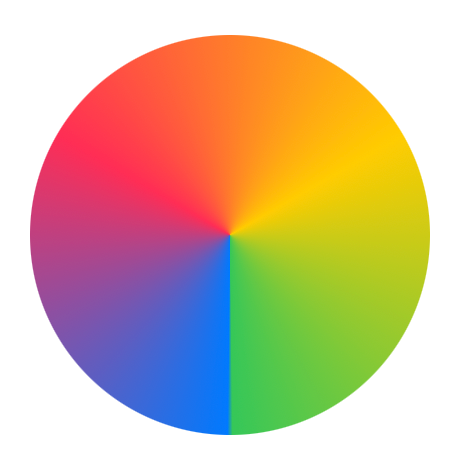This is the third and final article in my series on gradients in SwiftUI. Previously  I published a couple of following articles on SwiftUI gradients.

In today's article, we will learn what angular gradients are and how to use them in SwiftUI with examples and code samples.

Apple defines angular gradient as follows,

An angular gradient is also known as a “conic” gradient. This gradient applies the color function as the angle changes, relative to a center point and defined start and end angles

As suggested by the above definition, angular gradient always moves in the radial direction with reference to a pre-defined center. It always appears in a 360-degree angle space with no gaps. Any gaps, if present are filled with the first or last colors defined in the `gradient` parameter for the angular gradient.

## How to define Angular Gradient in SwiftUI

Developers can simply use `AngularGradient` API to define the angular gradient. This API looks like this,

``````
public init(gradient: Gradient, center: UnitPoint, startAngle: Angle = .zero, endAngle: Angle = .zero)

``````

Gradient initializer takes the following parameters in its initializer,

• `gradient` - Defines colors used in the angular gradient
• `center` - The gradient center relative to the container to which it is added. For example, it could either be the container center or its top-left or top-right corner. If the gradient is shifted to a center other than the default center, its appearance will vary accordingly
• `startAngle` - Angle at which gradient should begin
• `endAngle` - Angle at which gradient should end

We will look at the examples below to see how gradient appearance changes as we tweak these input parameters.

### Creating a Simple Angular Gradient

For the simplest case, let's make an angular gradient containing 4 colors. We will use it to fill the square of fixed dimensions and also clip it in a circular shape so that the square length becomes the circle diameter.

``````

``````

As you can see, it starts at 0 degrees angle, and ends at the same spot and 4 colors are equally divided in the given angular space inside a rectangular container shaped like a circle.

### Changing the Center

By default, the gradient center is same as the container. However, we can change it to be anywhere relative to the container. For simple cases, we will position it on a couple of container corners. To stress this point, we will use the rectangular container to make it easier to see where exactly the gradient center lies.

``````

``````

As you can see, as we change the center, the gradient center is fixated on that point, and the rest of the rectangle area is filled by extending available colors in the opposite direction of the center. The part of the gradient not visible in the space above lies outside of the container and thus is not drawn.

### Changing the Start and End Angles

Next, we will see how changing star and end angles affect the gradient appearance. By default angular gradient starts at 0 degrees and ends at 360 degrees angle spreading all available colors equally in the given radial space.

### When `endAngle - startAngle < 360` degrees

If we change the start angle, the gradient starts at a different angle relative to 0 degrees.

``````

``````Left with a gradient start at an angle of 90 while on the right with a start angle of 180 degrees

You can also see how it changes the gradient composition. If the start angle is moved forward while keeping the end angle unchanged, our gradients have less space and thus are non-evenly spread.

As Apple documentation states,

If endAngle - startAngle < 2π, the gradient fills the missing area with the colors defined by gradient locations one and zero

In our cases above, since we have shifted the start angle by more than 0 degrees, the difference between start and end angle is less than 360 and thus it leaves the blank space which is filled by the first and last colors - In this case blue and green colors which gets the highest percentage of space in the gradient.

#### Effects of changing start angle and end angle on angular gradients

This effect is even more pronounced with another example,

``````

``````

In the above code, the gradient starts at 90 degrees and ends at 270 degrees. This leaves only 180 degrees area for the gradient to fill in. Rest of the area lying between the start and end colors is equally filled in by them - In this case by green and blue colors

Output:

If we need to keep the same composition for all colors while changing the start angle, we need to increase the end angle by the same value. This results in a rotation effect where everything remains the same except the new gradient now appears rotated.

``````
// Rotated by 90 degrees

// Rotated by 180 degrees

``````On left, rotated by 90 degrees while on right rotated by 180 degrees

### When `endAngle - startAngle > 360` degrees

The gradient reacts in a different way when the difference between the end angle and start angle is > 360. As official Apple documentation states,

If `endAngle - startAngle > 2π`, the gradient only draws the last complete turn

What it means is, even if you assign > 360 space to color gradients, it can only fit colors in the 360 angular space. If it overflows, it only shows the last X% of the total gradient in the given 360 degrees space.

For example, let's say you create a 4-color angular gradient in angular space ranging from 0-540 degrees angle. However, there is only 0-360 degrees space on the circle, so it will chop the first 180 and will only show the last 360 degrees part on the gradient.

In other words, each color gets 25% of 540 angles, that is 135 degrees and since 360/135 = 2.67, only the last 2 colors and 0.67 percent of the third-last color are shown on the gradient,

``````

``````

As evident from this screenshot, the first color - blue is completely invisible. It exists somewhere, but it's not visible due to insufficient space.

If we want to see blue color, we need to reduce the end angle. If we make it 440, it gives just enough space for the blue color to become visible on the gradient.

``````

``````
💡
Please note that all the angles used in this post were positive angles that caused the angular gradient to move in the clockwise direction. If we had used negative angles, it would've moved the angular gradient in the counter-clockwise direction

As we already saw in the above examples, gradients can be used with `fill` API on shapes to fill their area with gradient content. All the gradients conform to `ShapeStyle` and `View` protocols so they can be used in any function arguments that take these protocol types as arguments.

Similarly, gradients can be used with `background` API to style backgrounds.

``````
Text("Text")
.frame(maxWidth: .infinity, maxHeight: .infinity)

``````

Or even with a stroke API to decorate the border of the content.

``````

Circle()
.stroke(